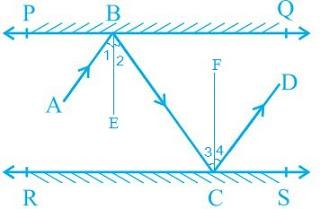Guru

# In Fig. 6.33, PQ and RS are two mirrors placed parallel to each other. An incident ray AB strikes the mirror PQ at B, the reflected ray moves along the path BC and strikes the mirror RS at C and again reflects back along CD. Prove that AB CD. Q.6

• 0

what is the tricky way for solving the question of class 9th ncert math of Lines and Angles chapter of ncert of exercise 6.2of math give me the best and simple way for solving this question in easy and simple way In Fig. 6.33, PQ and RS are two mirrors placed parallel to each other. An incident ray AB strikes the mirror PQ at B, the reflected ray moves along the path BC and strikes the mirror RS at C and again reflects back along CD. Prove that AB CD.

Share

1. First, draw two lines BE and CF such that BE ⊥ PQ and CF ⊥ RS.

Now, since PQ RS,

So, BE CFWe know that,

Angle of incidence = Angle of reflection (By the law of reflection)

So,

1 = 2 and

3 = 4

We also know that alternate interior angles are equal. Here, BE ⊥ CF and the transversal line BC cuts them at B and C

So, 2 = 3 (As they are alternate interior angles)

Now, 1 +2 = 3 +4

Or, ABC = DCB

So, AB CD alternate interior angles are equal)

• 0